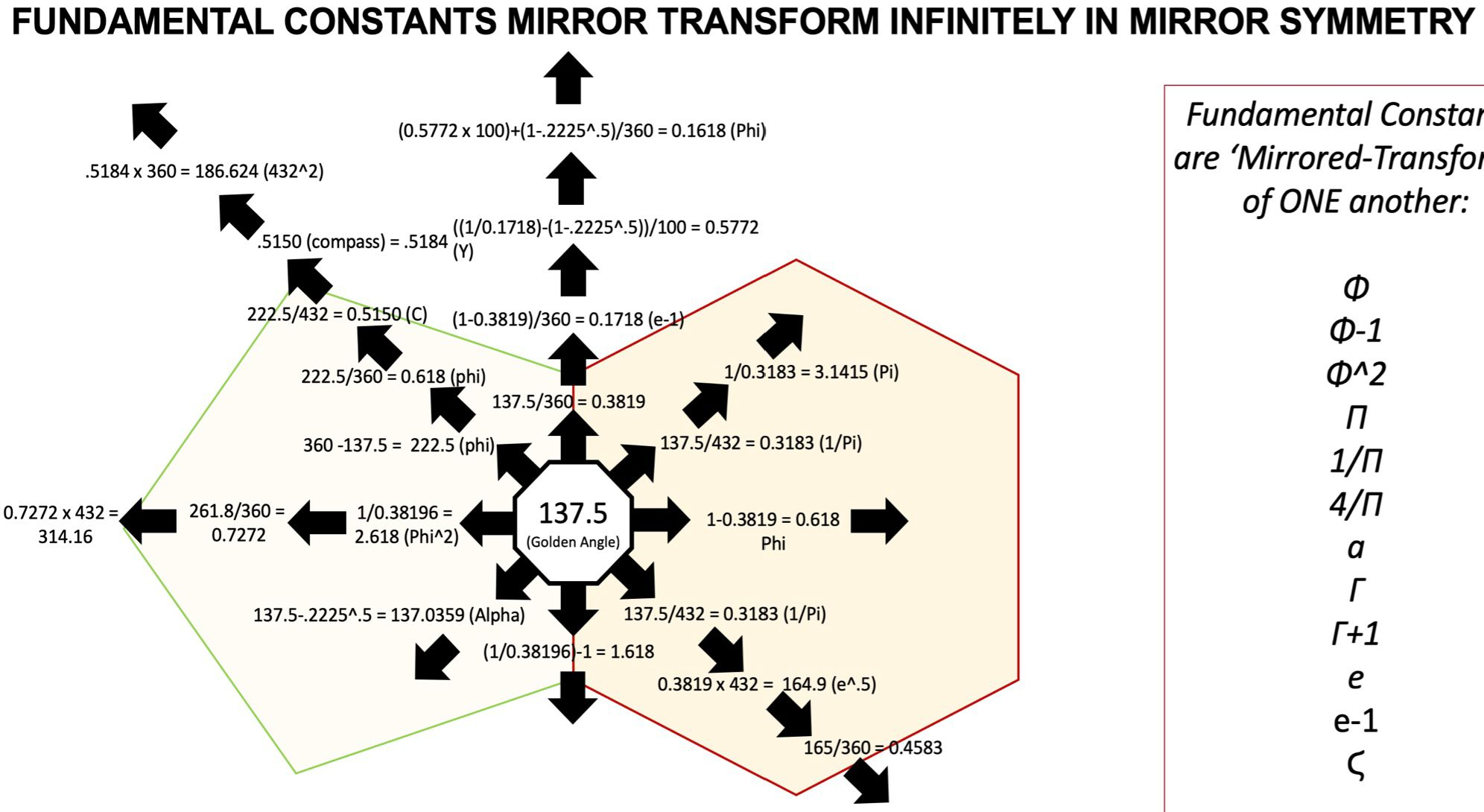Are ALL mathematical and physical constants simply mirrored interchangeable transformations emanating from a single constant: The Golden Angle (137.51°)?

137.51° / 360° = .3819; 1-.3819 = .618 = φ (The Golden Ratio) (φ360° = 222.49°)

618° / 360° = 1.718; 1 + 1.718 = 2.718 = e (The Euler Number)

(1/.1718 – (1-.22249^.5))/10^2 = .5772 = γ (The Euler Mascheroni Constant)

137.51° / 360° = .3819; 1/.3819 = 2.618 = Φ^2 (The Golden Ratio Squared)

Φ^2 * 1.2 = π. (Pi) This means that Φ^2 is actually π toggling between Base10 and Base12 numbering systems.

1/(π-1) = .4669 = σ (Feigenbaum Constant which determines all population growth)

1.3748^π = e

10^4/e^π = 432

432*1.2 = 518.4; .5184*360° = 186.624° = C (speed of Light in miles/sec) 432^2 = 186,624

(π^2)/6 = ζ (Riemann Zeta Function which governs prime number distribution

360° – 137.51° = 222.49°; 222.49° / 360° = φ; 137.51° – (.22249)^.5 = 137.0359 = α^-1 (Fine Structure Constant)

(α^-1) / 360° = .380649; 1 + .380649 = K (The Boltzmann Constant which defines the relationship between Temperature and Kinetic Energy)

The above demonstrates how the 10 most fundamental mathematical and physical ‘constants’ are all approximately interchangeable (Φ,π,e,γ,A°,σ,ζ,α,C, K etc…) AND YET…..we perceive them all as separate and entirely unrelated one to each other…..

Or, is this perhaps a metaphor for how we perceive each other….all the distinct and different individuals that populate our world? Are they truly separate from us or like the constants above, simply mirrored transformations emanating from the same ONE…..

Some food for thought this Tuesday morning in August (which btw means ‘Great, Magnificent and Majestic’, quite befitting of Leo ♌️ the Lion 🦁).